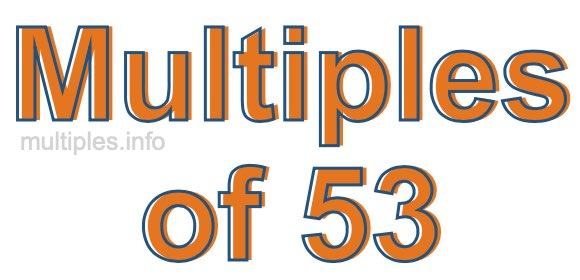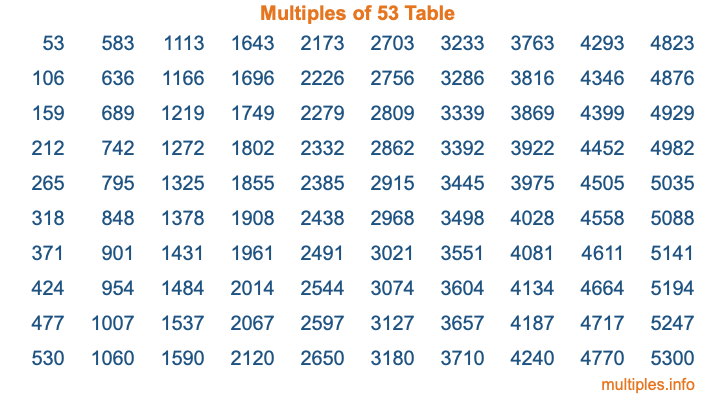Multiples of 53Welcome to the Multiples of 53 page. Here we will first teach you everything you will ever need to know about the multiples of 53, and then give you a study guide summary of everything we taught you to make sure you remember it all. Use this page to look up facts and learn information about the multiples of 53. This page will make you a multiples of fifty-three expert!

Definition of Multiples of 53
Multiples of 53 are all the numbers that when divided by 53 equal an integer. Each of the multiples of 53 are called a multiple. A multiple of 53 is created by multiplying 53 by an integer.

Therefore, to create a list of multiples of 53, you start with 1 multiplied by 53, then 2 multiplied by 53, then 3 multiplied by 53, and so on for as long as you want. Thus, the list of the first five multiples of 53 is 53, 106, 159, 212, and 265. To see a larger list of multiples of 53, see the printable image of Multiples of 53 further down on this page. We also have a category where you can choose any nth multiple of 53.

Multiples of 53 Checker
The Multiples of 53 Checker below checks to see if any number of your choice is a multiple of 53. In other words, it checks to see if there is any number (integer) that when multiplied by 53 will equal your number. To do that, we divide your number by 53. If the the quotient is an integer, then your number is a multiple of 53.

Is  a multiple of 53?

Least Common Multiple of 53 and ...
A Least Common Multiple (LCM) is the lowest multiple that two or more numbers have in common. This is also called the smallest common multiple or lowest common multiple and is useful to know when you are adding our subtracting fractions. Enter one or more numbers below (53 is already entered) to find the LCM.

Check out our LCM Calculator if you need more details about the Least Common Multiple or if you need the LCM for different numbers for adding and subtraction fractions.

nth Multiple of 53
As we stated above, 53 is the first multiple of 53, 106 is the second multiple of 53, 159 is the third multiple of 53, and so on. Enter a number below to find the nth multiple of 53.

th multiple of 53

Multiples of 53 vs Factors of 53
53 is a multiple of 53 and a factor of 53, but that is where the similarities end. All postive multiples of 53 are 53 or greater than 53. All positive factors of 53 are 53 or less than 53.

Below is the beginning list of multiples of 53 and the factors of 53 so you can compare:

Multiples of 53: 53, 106, 159, 212, 265, etc.

Factors of 53: 1, 53

As you can see, the multiples of 53 are all the numbers that you can divide by 53 to get a whole number. The factors of 53, on the other hand, are all the whole numbers that you can multiply by another whole number to get 53.

It's also interesting to note that if a number (x) is a factor of 53, then 53 will also be a multiple of that number (x).

Multiples of 53 vs Divisors of 53
The divisors of 53 are all the integers that 53 can be divided by evenly. Below is a list of the divisors of 53.

Divisors of 53: 1, 53

The interesting thing to note here is that if you take any multiple of 53 and divide it by a divisor of 53, you will see that the quotient is an integer.

Multiples of 53 Table
Below is an image of the first 100 multiples of 53 in a table. The table is in chronological order, column by column. The first column has the first ten multiples of 53, the second column has the next ten multiples of 53, and so on.The Multiples of 53 Table is also referred to as the 53 Times Table or Times Table of 53. You are welcome to print out our table for your studies.

Negative Multiples of 53
Although not often discussed or needed in math, it is worth mentioning that you can make a list of negative multiples of 53 by multiplying 53 by -1, then by -2, then by -3, and so on, to get the following list of negative multiples of 53:

-53, -106, -159, -212, -265, etc.

Multiples of 53 Summary
Below is a summary of important Multiples of 53 facts that we have discussed on this page. To retain the knowledge on this page, we recommend that you read through the summary and explain to yourself or a study partner why they hold true.

There are an infinite number of multiples of 53.

A multiple of 53 divided by 53 will equal a whole number.

53 divided by a factor of 53 equals a divisor of 53.

The nth multiple of 53 is n times 53.

The largest factor of 53 is equal to the first positive multiple of 53.

53 is a multiple of every factor of 53.

53 is a multiple of 53.

A multiple of 53 divided by a divisor of 53 equals an integer.

53 divided by a divisor of 53 equals a factor of 53.

Any integer times 53 will equal a multiple of 53.

Multiples of a Number
Here you can get the multiples of another number, all with the same attention to detail as we did for multiples of 53 on this page.

Multiples of
Multiples of 54
Did you find our page about multiples of fifty-three educational? Do you want more knowledge? Check out the multiples of the next number on our list!